Processing ......FreeComputerBooks.com Links to Free Computer, Mathematics, Technical Books all over the World

Model Theory
🌠 Top Free Programming Books - 100% Free or Open Source!
• Title Model Theory
• Authors C. Ward Henson
• Publisher: University of Illinois at Urbana-Champaign
• Paperback: N/A
• eBook: PDF
• Language: English
• ISBN-10: N/A
• ISBN-13: N/A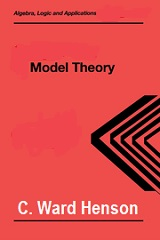Book Description

This is an up-to-date textbook of model theory taking the reader from first definitions to Morley's theorem and the elementary parts of stability theory.

It introduces the model theory of first-order logic, avoiding syntactical issues not too relevant to model theory. In this spirit, the compactness theorem is proved via the algebraically useful ultrsproduct technique (rather than via the completeness theorem of first-order logic). This leads fairly quickly to algebraic applications, like Malcev's local theorems of group theory and, after a little more preparation, to Hilbert's Nullstellensatz of field theory.

Besides standard results such as the compactness and omitting types theorems, it also describes various links with algebra, including the Skolem-Tarski method of quantifier elimination, model completeness, automorphism groups and omega-categoricity, ultraproducts, O-minimality and structures of finite Morley rank.

• C. Ward Henson is a Professor Emeritus at University of Illinois at Urbana-Champaign.
Reviews, Ratings, and Recommendations: Related Book Categories: Read and Download Links:Similar Books:
•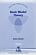Basic Model Theory (Kees Doets)

As the title indicates, this book introduces the reader to what is basic in model theory. A special feature is its use of the Ehrenfeucht game by which the reader is familiarised with the world of models.

•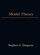Model Theory (Stephen G. Simpson)

This concise introduction to model theory begins with standard notions and takes the reader through to more advanced topics such as saturated and prime models. Concrete mathematical examples are included throughout to make the concepts easier to follow.

•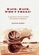Hack, Hack, Who's There? A Gentle Introduction to Model Theory

The skeleton of this narrative nonfiction book is a science fiction story without the usual mangling of physics. The flesh is composed of self-contained non-technical mainstream explanations and examples of the field of mathematics called Model Theory.

•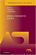Model-Theoretic Logics (Jon Barwise, et al)

This book brings together several directions of work in model theory between the late 1950s and early 1980s. It provides an introduction to the subject as a whole, as well as to the basic theory and examples. Many chapters can be read independently.

•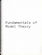Fundamentals of Model Theory (William Weiss, et al)

This book is a concluding discussion focuses on the relationship between proofs and formal derivations, and the role proofs may play as part of a general theory of evidence. It is a primer which will give someone a self contained overview of the subject.

•Model Theory, Algebra, and Geometry (Deirdre Haskell, et al)

Tis book gives the necessary background for understanding both the model theory and the mathematics behind the apps, begins with an introduction to model theory, broadens into three components: pure model theory, geometry, and the model theory of fields.

•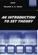An Introduction to Set Theory (William A. R. Weiss)

This book covers the basics: relations, functions, orderings, finite, countable, and uncountable sets, and cardinal and ordinal numbers, gives students sufficient grounding in a rigorous approach to the revolutionary results of set theory.

Book Categories
 :All CategoriesTop Free BooksRecent BooksMiscellaneous BooksComputer EngineeringComputer LanguagesComputer ScienceData Science/DatabasesJava and Java EE (J2EE)Linux and UnixMathematicsMicrosoft and .NETMobile ComputingNetworking and CommunicationsSoftware EngineeringSpecial TopicsWeb Programming
Other Categories